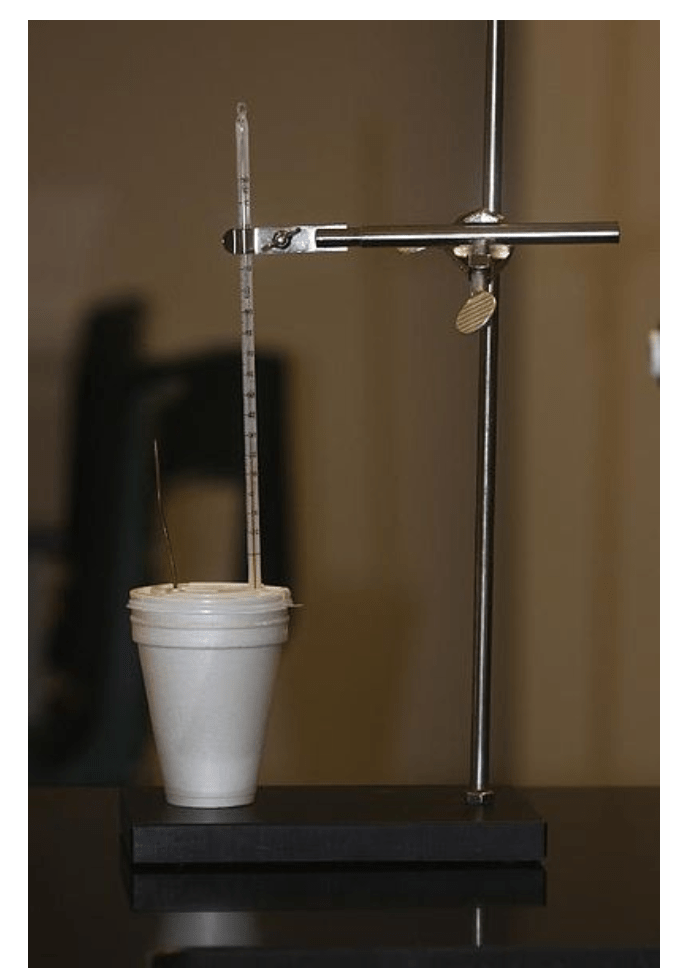Cyber Sale - Only 3 Days Remaining: ALL MCAT Products & Admissions Services

MCAT Content / Energy Changes In Chemical Reactions / Measure Of Heat Changes Calorimetry Heat Capacity Specific Heat

### Measurement of Heat Changes (calorimetry), Heat Capacity, Specific Heat

Topic: Energy Changes In Chemical Reactions

Calorimetry is the study of transferring energy via heat, which is energy transferred from the result of a temperature change.

Heat can be transferred in several ways. In solids, it moves via conduction by direct contact, convection in liquids and gases as a movement of particles or by radiation where heat is transferred by electromagnetic radiation and does not require matter.

The study of heat transfer is calorimetry, where measurements can be made of the heat energy transferred from one substance to another. A calorimeter measures the change in enthalpy of a reaction. The heat transferred to/from the solution in order for the reaction to occur is equal to the change in enthalpy. It is assumed that the volume and pressure of the system are constant. A simple example of a calorimeter is a coffee-cup calorimeter, which is constructed from two nested Styrofoam cups and a lid with two holes, which allows for the insertion of a thermometer and a stirring rod. The inner cup holds a known amount of a liquid, usually water, that absorbs the heat from the reaction.The energy in a system can be transferred throughout via heat transfer, which is described as heat. This relationship, with no other forms of energy transfer involved, can be represented by ΔU=q, where q represents thermal energy in Joules, J.

The relationship between Joules and calories is 1 calorie = 4.184 J.

Give the temperature change of a system, the energy transferred as heat can be calculated using the following formula:

q = mCΔT

Where is the thermal energy in J, C is the heat capacity, and ΔT is the temperature change measured in K, and m is the mass of the substance being heated.

The variable C, heat capacity is an intrinsic physical property of a substance that measures the amount of heat required to change that substance’s temperature by a given amount. In the International System of Units (SI), heat capacity is expressed in units of joules per kelvin. There are two derived quantities that specify heat capacity as an intensive property (i.e., independent of the size of a sample) of a substance. They are specific heat capacity and molar heat capacity. Specific heat capacity is substance-specific and can be found in literature or calculated if all other values in the equation are given; the unit for the specific heat capacity is in Joules per Kelvin per gram, or J/(K*g) (also can be written as J/(°C*g). Molar heat capacity is the same concept but gives the heat capacity per moles rather than per grams. For example, the specific heat capacity of water is 4.18 J/(K*g), and the molar heat capacity is 75 J/(K*mol)

Practice Questions

Heat engines and work

MCAT Official Prep (AAMC)

Chemistry Question Pack Passage 5 Question 26

Sample Test C/P Section Passage 7 Question 36

Sample Test C/P Section Question 59

Practice Exam 4 C/P Section Question 59

Key Points

• 1 calorie = 4.18 Joules

• Change in enthalpy can be calculated based on the change in temperature of the solution, its specific heat capacity, and mass.

• The thermal energy given in Joules from a temperature change can be calculated using q = mCΔT

• Calorimeters can be used to measure the change in heat energy

Key Terms

Specific heat capacity: heat capacity divided by the mass of a given substance

Molar heat capacity: heat capacity divided by the moles of a given substance

Heat: transfer of energy that results in the change of temperature

Temperature: a measure of the thermal energy in a system

Billing Information